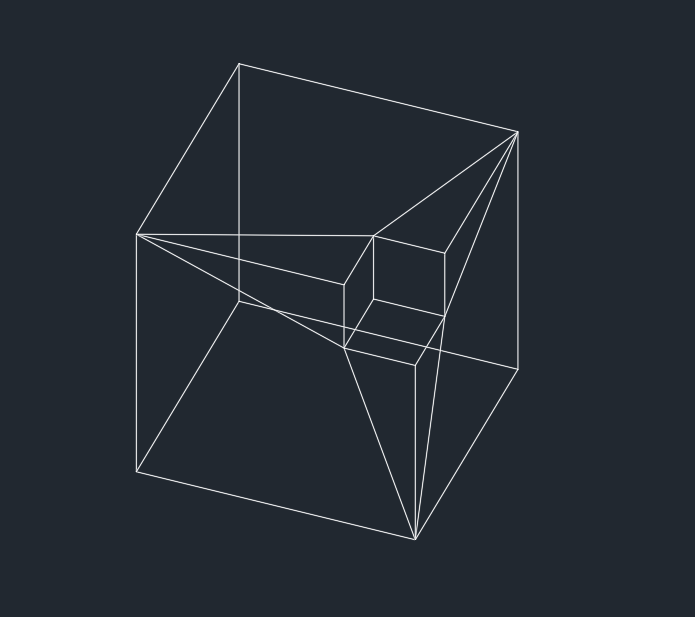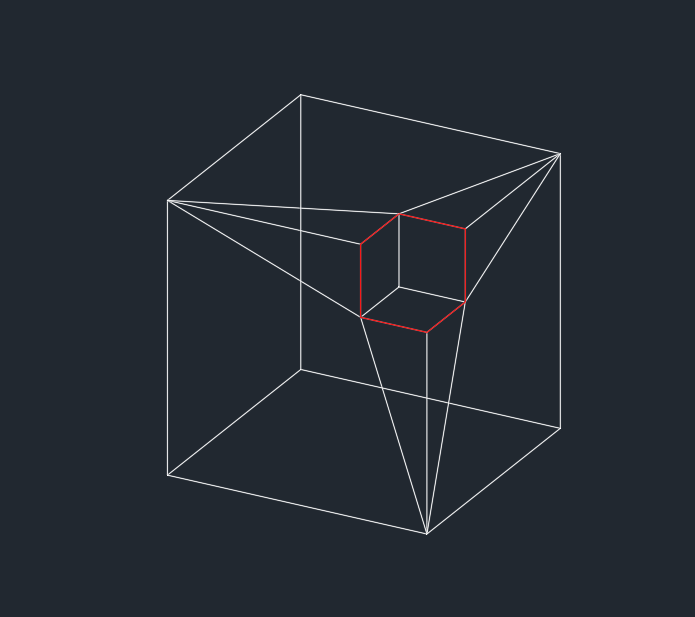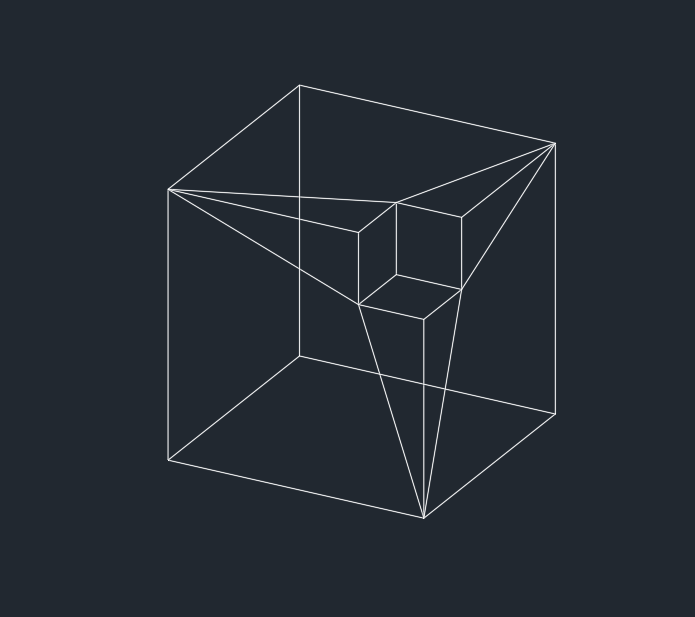# Smoothing meshes

## Alexandr Bryzgalin

January 16, 2020

OdDbSubDMeshImpl is a class that has a set of methods used for smoothing. In general, a mesh can be smoothed using these methods:

``````OdResult subdDivideUp ();
OdResult subdDivideDown ();``````

The first method pushes the smoothness level up one by increasing the subDvision level. The second method drops the smoothness level down one by decreasing the subDvision level.

But these methods only set the smoothness level, and changes are applied by calling OdResult subdRefine (), which recalculates mesh data:

So the code will take the form of:

``````…
mesh.subdDivideUp();
mesh.subdRefine();
...``````

Or you can set the smoothness level directly by calling:

``OdResult subdLevel (OdInt32& result)``

The image below shows how changing the smoothness can affect a sample solid model. Here the smoothness level is increased from 0 to 3 by 1 and after that decreases from 3 to 0.In the image above, you can see a visual representation of the smoothness level, as well as how it is affected by subdDivideUp () and subdDivideDown (). After, when subdRefine () is called, the faces, edges and vertices are recomputed.

At level 0, there is an unmodified entity. With smoothness level 3, additional faces are calculated according to the original faces, edges, and vertices. Thus, we obtain smoothness level 1. Next, the calculations are repeated, but already the data obtained smoothness level 1, thus obtains smoothness level 2 and so on. As a result, this makes it possible to smooth the mesh. Moreover, all this calculated data for this smoothness level is virtual; it is not written to the file and is recalculated at rendering, proceeding from the set smoothness level.

Also you can control the smoothing of subentities. What does this mean? Let's say we have a mesh and we want to smooth some of the edges. In the following image, the smoothed edges are marked in red.You can use the following:

``````OdResult setCrease (double creaseVal);
OdResult setCrease (const OdDbFullSubentPathArray & subentPaths, double creaseVal);``````

With these methods, you can make a face, edge, or vertex of the mesh object "sharp." This allows you to indicate which sub-objects of the mesh object are not affected by the smoothing scheme.

These methods accept creaseVal, which can be equal to:

• -1 — The sub-entity is not smoothed.
• 0 — The sub-entity is always smoothed.
• Any positive value — The sub-entity is smoothed only if this value is less than or equal to the smoothness level of the mesh. In other words, this value indicates the level at which smoothing should begin for a given sub-entity. Currently this option supports only edges; faces and vertices are not supported yet.

For example, if you call setCrease (1.); for an edge sub-entity (see the previous image) and change the mesh smoothness level from 0 to 3, edges are smoothed only if the smoothness level is 1 (see image below).